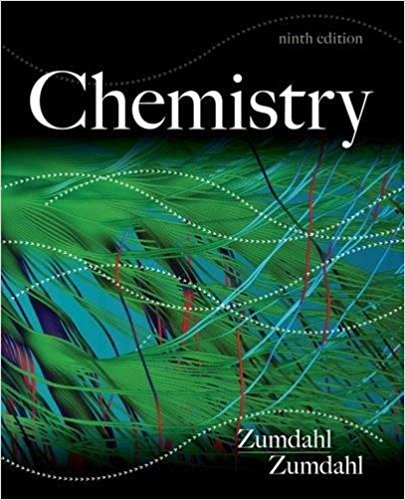### Solution Found!# Understanding Microwaves: Boiling Water's Time and Photon Count

Chapter 7, Problem 138

(choose chapter or problem)

Get Unlimited Answers! Check out our subscriptions
QUESTION:

A certain microwave oven delivers 750. watts (J/s) of power to a coffee cup containing 50.0 g of water at $$25^\circ C$$. If the wavelength of microwaves in the oven is 9.75 cm, how long does it take, and how many photons must be absorbed, to make the water boil? The specific heat capacity of water is $$4.18\ J^{\circ}/C\cdot g$$. Assume that only the water absorbs the energy of the microwaves.

##### Understanding Microwaves: Boiling Water's Time and Photon CountWant To Learn More? To watch the entire video and ALL of the videos in the series:

This video breaks down the process of calculating the time and number of photons required to boil water in a microwave. Using given power, wavelength, and water specifics, we determine both energy needs and photon count.

QUESTION:

A certain microwave oven delivers 750. watts (J/s) of power to a coffee cup containing 50.0 g of water at $$25^\circ C$$. If the wavelength of microwaves in the oven is 9.75 cm, how long does it take, and how many photons must be absorbed, to make the water boil? The specific heat capacity of water is $$4.18\ J^{\circ}/C\cdot g$$. Assume that only the water absorbs the energy of the microwaves.

Step 1 of 4

Coffee cup calorimeter

A calorimeter is an analytical setup to study the energy changes associated with different processes. In the coffee cup calorimeter, the temperature change is used to calculate the energy change using the formula:

$$q = mc \ \Delta T$$

Where m is the mass taken, c is the specific heat, and $$\Delta T$$ represents temperature change.